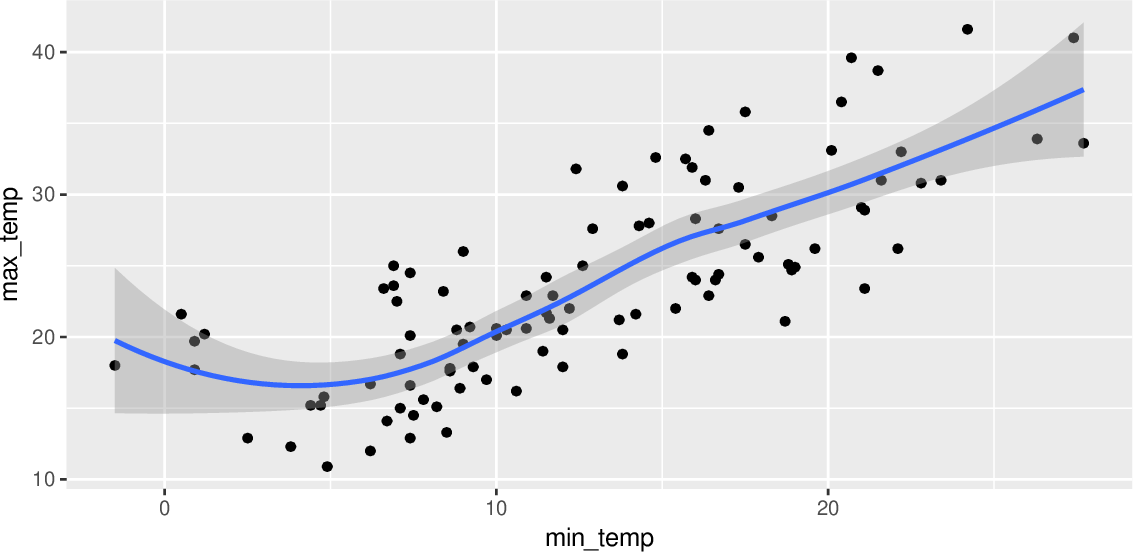Data Science Desktop Survival Guide by Graham WilliamsDesktop Survival Project Home Preface Data Science Introducing R R Constructs R Tasks R Strings R Read, Write, and Create Data Template Data Exploration Data Wrangling Data Visualisation Statistics ML Template ML Scenarios ML Activities ML Applications ML Algorithms Cluster Analysis Decision Trees Computer Vision Graph Data Privacy Literate Data Science Coding with Style Resources Bibliography Index

## Scatter Plot Smooth Loess

20180603set.seed(26439) ds %>%   sample_n(100) %>%   ggplot(aes(x=min_temp, y=max_temp)) +   geom_point() +   stat_smooth(method="loess", formula=y~x) A smooth curve here fits the statistical loess function for a single smoother line to the points. The resulting plot uses ggplot2::geom_smooth() with a so called locally weighted scatterplot smoothing method or LOESS to produce the smoothed line. As a result we can observe statistically something of a relationship between the two variables although it is not a simple relationship.